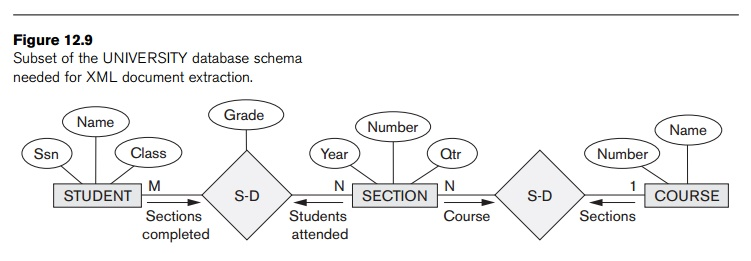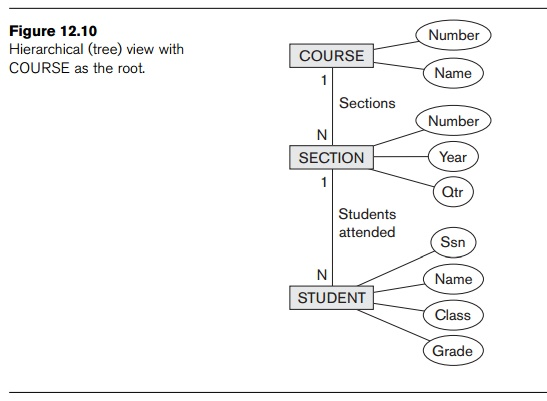Home | | Database Management Systems | Extracting XML Documents from Relational Databases

# Extracting XML Documents from Relational Databases

1. Creating Hierarchical XML Views over Flat or Graph-Based Data 2. Breaking Cycles to Convert Graphs into Trees 3. ther Steps for Extracting XML Documents from Databases

Extracting XML Documents from Relational Databases

1. Creating Hierarchical XML Views over Flat or Graph-Based Data

This section discusses the representational issues that arise when converting data from a database system into XML documents. As we have discussed, XML uses a hierarchical (tree) model to represent documents. The database systems with the most widespread use follow the flat relational data model. When we add referential integrity constraints, a relational schema can be considered to be a graph structure (for example, see Figure 3.7). Similarly, the ER model represents data using graph-like structures (for example, see Figure 7.2). We saw in Chapter 9 that there are straightforward mappings between the ER and relational models, so we can conceptually represent a relational database schema using the corresponding ER schema. Although we will use the ER model in our discussion and examples to clarify the conceptual differences between tree and graph models, the same issues apply to converting relational data to XML.

We will use the simplified UNIVERSITY ER schema shown in Figure 12.8 to illustrate our discussion. Suppose that an application needs to extract XML documents for student, course, and grade information from the UNIVERSITY database. The data needed for these documents is contained in the database attributes of the entitytypes COURSE, SECTION, and STUDENT from Figure 12.8, and the relationships S-S and C-S between them. In general, most documents extracted from a database will only use a subset of the attributes, entity types, and relationships in the database. In this example, the subset of the database that is needed is shown in Figure 12.9.

At least three possible document hierarchies can be extracted from the database subset in Figure 12.9. First, we can choose COURSE as the root, as illustrated in Figure 12.10. Here, each course entity has the set of its sections as subelements, and each section has its students as subelements. We can see one consequence of modeling the information in a hierarchical tree structure. If a student has taken multiple sections, that student’s information will appear multiple times in the document— once under each section. A possible simplified XML schema for this view is shown in Figure 12.11. The Grade database attribute in the S-S relationship is migrated to the STUDENT element. This is because STUDENT becomes a child of SECTION in this hierarchy, so each STUDENT element under a specific SECTION element can have a specific grade in that section. In this document hierarchy, a student taking more than one section will have several replicas, one under each section, and each replica will have the specific grade given in that particular section.Figure 12.11

XML schema document with course as the root.

<xsd:element name=“root”>

<xsd:sequence>

<xsd:element name=“course” minOccurs=“0” maxOccurs=“unbounded”>

<xsd:sequence>

<xsd:element name=“cname” type=“xsd:string” />

<xsd:element name=“cnumber” type=“xsd:unsignedInt” />

<xsd:element name=“section” minOccurs=“0” maxOccurs=“unbounded”>

<xsd:sequence>

<xsd:element name=“secnumber” type=“xsd:unsignedInt” />

<xsd:element name=“year” type=“xsd:string” />

<xsd:element name=“quarter” type=“xsd:string” />

<xsd:element name=“student” minOccurs=“0” maxOccurs=“unbounded”> <xsd:sequence>

<xsd:element name=“ssn” type=“xsd:string” /> <xsd:element name=“sname” type=“xsd:string” /> <xsd:element name=“class” type=“xsd:string” /> <xsd:element name=“grade” type=“xsd:string” />

</xsd:sequence>

</xsd:element>

</xsd:sequence>

</xsd:element>

</xsd:sequence>

</xsd:element>

</xsd:sequence>

</xsd:element>

In the second hierarchical document view, we can choose STUDENT as root (Figure 12.12). In this hierarchical view, each student has a set of sections as its child elements, and each section is related to one course as its child, because the relationship between SECTION and COURSE is N:1. Thus, we can merge the COURSE and SECTION elements in this view, as shown in Figure 12.12. In addition, the GRADE data-base attribute can be migrated to the SECTION element. In this hierarchy, the combined COURSE/SECTION information is replicated under each student who completed the section. A possible simplified XML schema for this view is shown in Figure 12.13.<xsd:element name=”root”> <xsd:sequence>

<xsd:element name=”student” minOccurs=”0” maxOccurs=”unbounded”> <xsd:sequence>

<xsd:element name=”ssn” type=”xsd:string” /> <xsd:element name=”sname” type=”xsd:string” /> <xsd:element name=”class” type=”xsd:string” />

<xsd:element name=”section” minOccurs=”0” maxOccurs=”unbounded”> <xsd:sequence>

<xsd:element name=”secnumber” type=”xsd:unsignedInt” /> <xsd:element name=”year” type=”xsd:string” /> <xsd:element name=”quarter” type=”xsd:string” /> <xsd:element name=”cnumber” type=”xsd:unsignedInt” /> <xsd:element name=”cname” type=”xsd:string” /> <xsd:element name=”grade” type=”xsd:string” />

</xsd:sequence>

</xsd:element>

</xsd:sequence>

</xsd:element>

</xsd:sequence>

</xsd:element>

Figure 12.13 XML schema document with student as the root.

The third possible way is to choose SECTION as the root, as shown in Figure 12.14. Similar to the second hierarchical view, the COURSE information can be merged into the SECTION element. The GRADE database attribute can be migrated to the STUDENT element. As we can see, even in this simple example, there can be numerous hierarchical document views, each corresponding to a different root and a different XML document structure.2. Breaking Cycles to Convert Graphs into Trees

In the previous examples, the subset of the database of interest had no cycles. It is possible to have a more complex subset with one or more cycles, indicating multiple relationships among the entities. In this case, it is more difficult to decide how to create the document hierarchies. Additional duplication of entities may be needed to represent the multiple relationships. We will illustrate this with an example using the ER schema in Figure 12.8.

Suppose that we need the information in all the entity types and relationships in Figure 12.8 for a particular XML document, with STUDENT as the root element. Figure 12.15 illustrates how a possible hierarchical tree structure can be created for this document. First, we get a lattice with STUDENT as the root, as shown in Figure 12.15(a). This is not a tree structure because of the cycles. One way to break the cycles is to replicate the entity types involved in the cycles. First, we replicate INSTRUCTOR as shown in Figure 12.15(b), calling the replica to the right INSTRUCTOR1. The INSTRUCTOR replica on the left represents the relationship between instructors and the sections they teach, whereas the INSTRUCTOR1 replica on the right represents the relationship between instructors and the department each works in. After this, we still have the cycle involving COURSE, so we can replicate COURSE in a similar manner, leading to the hierarchy shown in Figure 12.15(c). The COURSE1 replica to the left represents the relationship between courses and their sections, whereas the COURSE replica to the right represents the relationship between courses and the department that offers each course.In Figure 12.15(c), we have converted the initial graph to a hierarchy. We can do further merging if desired (as in our previous example) before creating the final hierarchy and the corresponding XML schema structure.

3. ther Steps for Extracting XML Documents from Databases

In addition to creating the appropriate XML hierarchy and corresponding XML schema document, several other steps are needed to extract a particular XML document from a database:

It is necessary to create the correct query in SQL to extract the desired information for the XML document.

Once the query is executed, its result must be restructured from the flat relational form to the XML tree structure.

The query can be customized to select either a single object or multiple objects into the document. For example, in the view in Figure 12.13, the query can select a single student entity and create a document corresponding to that single student, or it may select several—or even all—of the stu-dents and create a document with multiple students.

Study Material, Lecturing Notes, Assignment, Reference, Wiki description explanation, brief detail
Fundamentals of Database Systems : Object, Object-Relational, and XML: Concepts, Models, Languages, and Standards : XML: Extensible Markup Language : Extracting XML Documents from Relational Databases |